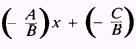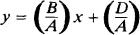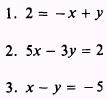Parallel and Perpendicular LinesCustom SearchPARALLEL AND PERPENDICULAR LINES The general equation of a straight line is often written with capital letters for coefficients, as follows: Ax+By+C=0 These literal coefficients, as they are called, represent the numerical coefficients encountered in a typical linear equation. Suppose we are given two equations that are duplicates except for the constant term, as follows: Ax +By+C=0 Ax +By+D=0 By placing these two equations in slope-intercept form, we can show that their slopes are equal, as follows: y =Thus, the slope of each line is -A/B. Since lines having equal slopes are parallel, we reach the follow�ing conclusion: In any two linear equations, if the coefficients of the x and y terms are identical in value and sign, then the lines represented by these equations are parallel. EXAMPLE: Write the equation of a line parallel to 3x - y - 2 = 0 and passing through the point (5,2). SOLUTION: The coefficients of x and y in the desired equa�tion are the same as those in the given equation. Therefore, the equation is 3x-y+D=0 Since the line passes through (5,2), the values x = 5 and y = 2 must satisfy the equation. Substituting these, we have 3(5) - (2) + D = 0 D= -13 Thus, the required equation is 3x-y- 13 =0 A situation similar to that prevailing with parallel lines involves perpendicular lines. For example, consider the equations Ax +By+C=0 Bx-Ay+D=0 Transposing these equations into the slope-intercept form, we have y =Since the slopes of these two lines are negative reciprocals, the lines are perpendicular. The conclusion derived from the foregoing discussion is as follows: If a line is to be perpendicular to a given line, the coefficients of x and y in the required equation are found by interchanging the coefficients of x and y in the given equation and changing the sign of one of them. EXAMPLE: Write the equation of a line perpendicular to the line x + 3y + 3 = 0 and having a y intercept of 5. SOLUTION: The required equation is 3x-y+D=0 Notice the interchange of coefficients and the change of sign. At the point where the line crosses the Y axis, the value of x is 0 and the value of y is 5. Therefore, the equation is 3(0) - (5) + D = 0 D=5 The required equation is 3x-y+5=0 1-19 PRACTICE PROBLEMS: 1. Find the equation of the line whose perpendicular forms an angle of 135' from the positive side of the X axis and whose perpendicular distance is V-2- units from the origin. Find the equations of the following lines: 2. Through (1,1) and parallel to 5x - 3y = 9. 3. Through (- 3,2) and perpendicular to x + y = 5. ANSWERS:Integrated Publishing, Inc. - A (SDVOSB) Service Disabled Veteran Owned Small Business